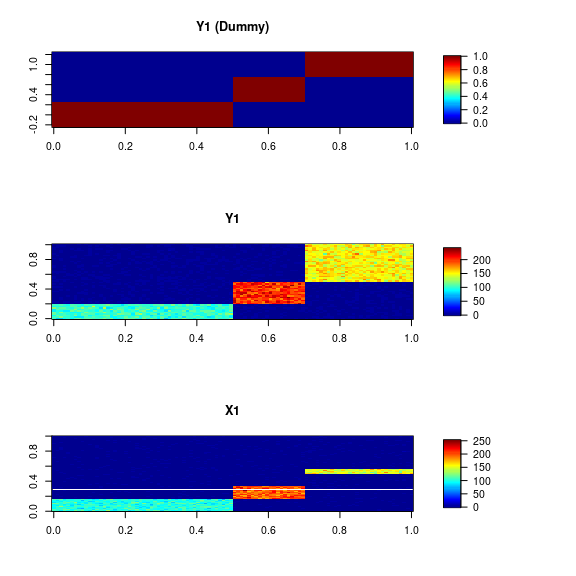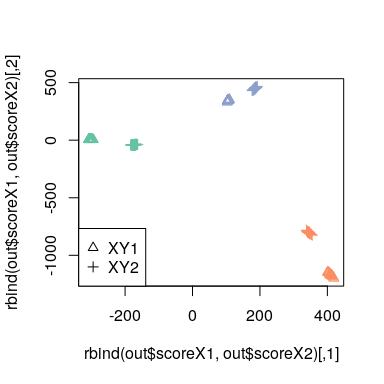# 2. Guided Partial Least Squares (guided-PLS)

Laboratory for Bioinformatics Research, RIKEN Center for Biosystems Dynamics Research
[email protected]

# Introduction

In this vignette, we consider a novel supervised dimensional reduction method guided partial least squares (guided-PLS).

Test data is available from toyModel.

library("guidedPLS")
data <- guidedPLS::toyModel("Easy")
str(data, 2)
## List of 8
##  $X1 : int [1:100, 1:300] 86 101 95 106 113 85 88 103 106 84 ... ##$ X2      : int [1:200, 1:150] 106 81 91 101 91 105 111 81 113 105 ...
##  $Y1 : int [1:100, 1:50] 101 77 77 87 101 89 111 113 101 112 ... ##$ Y1_dummy: num [1:100, 1:3] 1 1 1 1 1 1 1 1 1 1 ...
##  $Y2 : int [1:200, 1:50] 107 81 102 90 84 106 97 90 88 115 ... ##$ Y2_dummy: num [1:200, 1:3] 1 1 1 1 1 1 1 1 1 1 ...
##  $col1 : chr [1:100] "#66C2A5" "#66C2A5" "#66C2A5" "#66C2A5" ... ##$ col2    : chr [1:200] "#66C2A5" "#66C2A5" "#66C2A5" "#66C2A5" ...

You will see that there are three blocks in the data matrix as follows.

suppressMessages(library("fields"))
layout(c(1,2,3))
image.plot(data$Y1_dummy, main="Y1 (Dummy)", legend.mar=8) image.plot(data$Y1, main="Y1", legend.mar=8)
image.plot(data$X1, main="X1", legend.mar=8)# Guided Partial Least Squares (guided-PLS) Here, suppose that we have two data matrices $$X_1$$ ($$N \times M$$) and $$X_2$$ ($$S \times T$$), and the row vectors of them are assumed to be centered. Since these two matrices have no common row or column, integration of them is not trivial. Such a data structure is called “diagonal” and known as a barrier to omics data integration (Argelaguet 2021). Here is a simpler way to set up the problem; suppose that we have another set of matrices $$Y_1$$ ($$M \times I$$) and $$Y_2$$ ($$T \times I$$), which are the label matrices for $$X_1$$ and $$X_2$$, respectively. In guided-PLS, the data matrices $$X_1$$ and $$X_2$$ are projected into lower dimension via $$Y_1$$ and $$Y_2$$, and then PLS-SVD are performed against the $$Y_{1} X_{1}$$ and $$Y_{2} X_{2}$$ as follows: $\max_{W_{1},W_{2}} \mathrm{tr} \left( W_{1}^{T} X_{1}^{T} Y_{1}^{T} Y_{2} X_{2} W_{2} \right)\ \mathrm{s.t.}\ W_{1}^{T}W_{1} = W_{2}^{T}W_{2} = I_{K}$ ## Basic Usage guidedPLS is performed as follows. out <- guidedPLS(X1=data$X1, X2=data$X2, Y1=data$Y1, Y2=data$Y2, k=2) plot(rbind(out$scoreX1, out$scoreX2), col=c(data$col1, data$col2), pch=c(rep(2, length=nrow(out$scoreX1)), rep(3, length=nrow(out\$scoreX2))))
legend("bottomleft", legend=c("XY1", "XY2"), pch=c(2,3))# Session Information

## R version 4.3.0 (2023-04-21)
## Platform: x86_64-pc-linux-gnu (64-bit)
## Running under: Debian GNU/Linux bookworm/sid
##
## Matrix products: default
## LAPACK: /usr/lib/x86_64-linux-gnu/openblas-pthread/libopenblasp-r0.3.21.so;  LAPACK version 3.11.0
##
## locale:
##   LC_CTYPE=en_US.UTF-8       LC_NUMERIC=C
##   LC_TIME=en_US.UTF-8        LC_COLLATE=en_US.UTF-8
##   LC_MONETARY=en_US.UTF-8    LC_MESSAGES=en_US.UTF-8
##   LC_PAPER=en_US.UTF-8       LC_NAME=C
##  LC_MEASUREMENT=en_US.UTF-8 LC_IDENTIFICATION=C
##
## time zone: Etc/UTC
## tzcode source: system (glibc)
##
## attached base packages:
##  stats     graphics  grDevices utils     datasets  methods   base
##
## other attached packages:
##  fields_14.1       viridis_0.6.2     viridisLite_0.4.1 spam_2.9-1
##  guidedPLS_1.0.0
##
## loaded via a namespace (and not attached):
##   Matrix_1.5-3     gtable_0.3.3     jsonlite_1.8.4   highr_0.10
##   compiler_4.3.0   maps_3.4.1       gridExtra_2.3    jquerylib_0.1.4
##   scales_1.2.1     yaml_2.3.7       fastmap_1.1.1    lattice_0.20-45
##  ggplot2_3.4.2    R6_2.5.1         knitr_1.42       dotCall64_1.0-2
##  tibble_3.2.1     munsell_0.5.0    bslib_0.4.2      pillar_1.9.0
##  rlang_1.1.0      utf8_1.2.3       cachem_1.0.7     xfun_0.39
##  sass_0.4.5       cli_3.6.1        magrittr_2.0.3   digest_0.6.31
##  grid_4.3.0       irlba_2.3.5.1    lifecycle_1.0.3  vctrs_0.6.2
##  evaluate_0.20    glue_1.6.2       fansi_1.0.4      colorspace_2.1-0
##  rmarkdown_2.21   tools_4.3.0      pkgconfig_2.0.3  htmltools_0.5.5